##### Question

In: Statistics and Probability

# For a standard normal distribution, find: P(-1.64 < z < -1.48)

For a standard normal distribution, find:

P(-1.64 < z < -1.48)

## Solutions

##### Expert Solution

We can solve this using two methods and using two different types of standard normal tables.

P(-1.64 < z < -1.48)

METHOD 1:

For finding P(-1.64 < z < -1.48)

we can use the standard normal table in which entries are negatives z or can be said as the entries which cover the left side of the curve which is less than the center of the curve.

The area under the curve areas: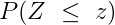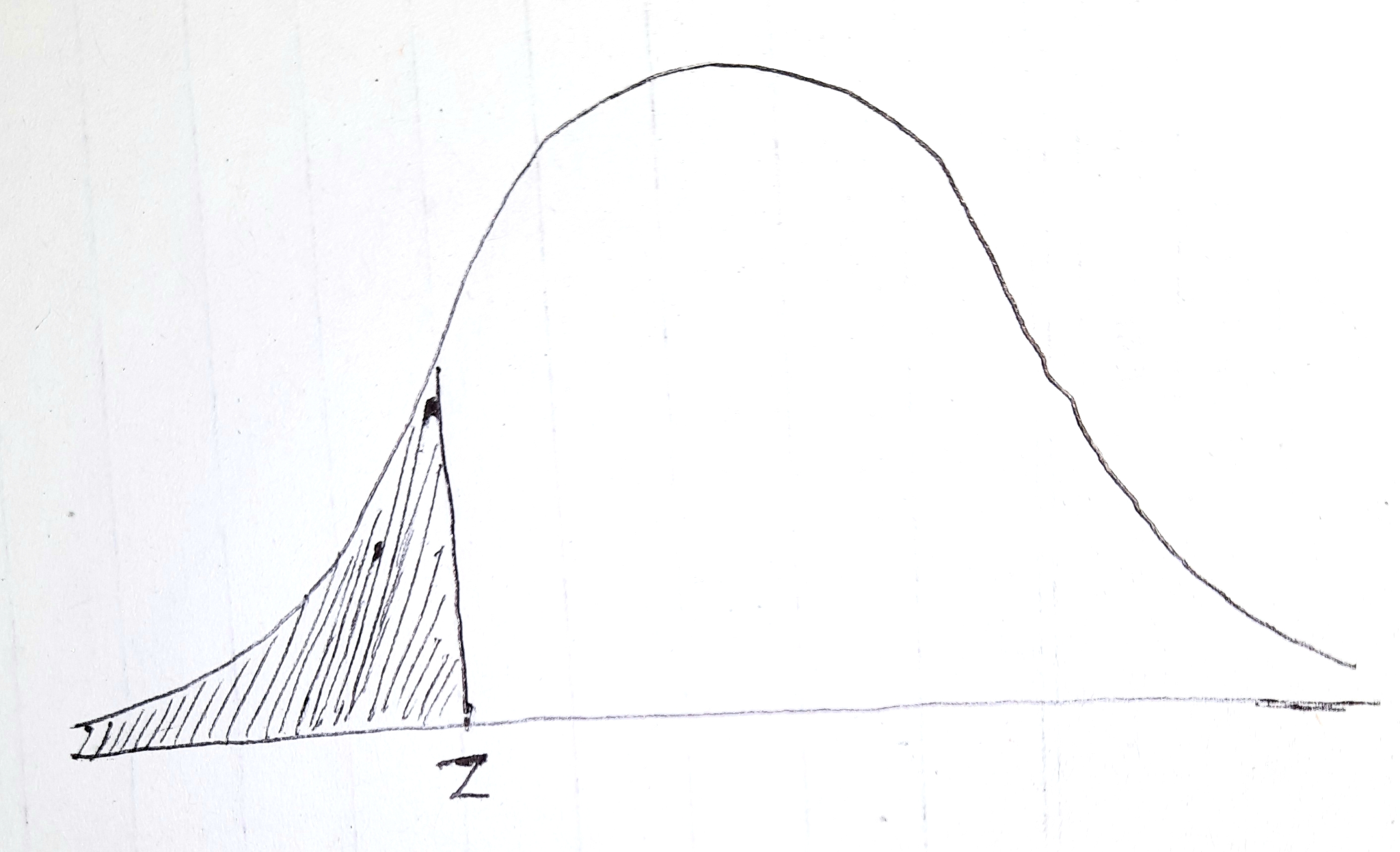Thus, P(-1.64 < z < -1.48) can be written as: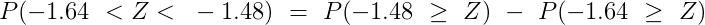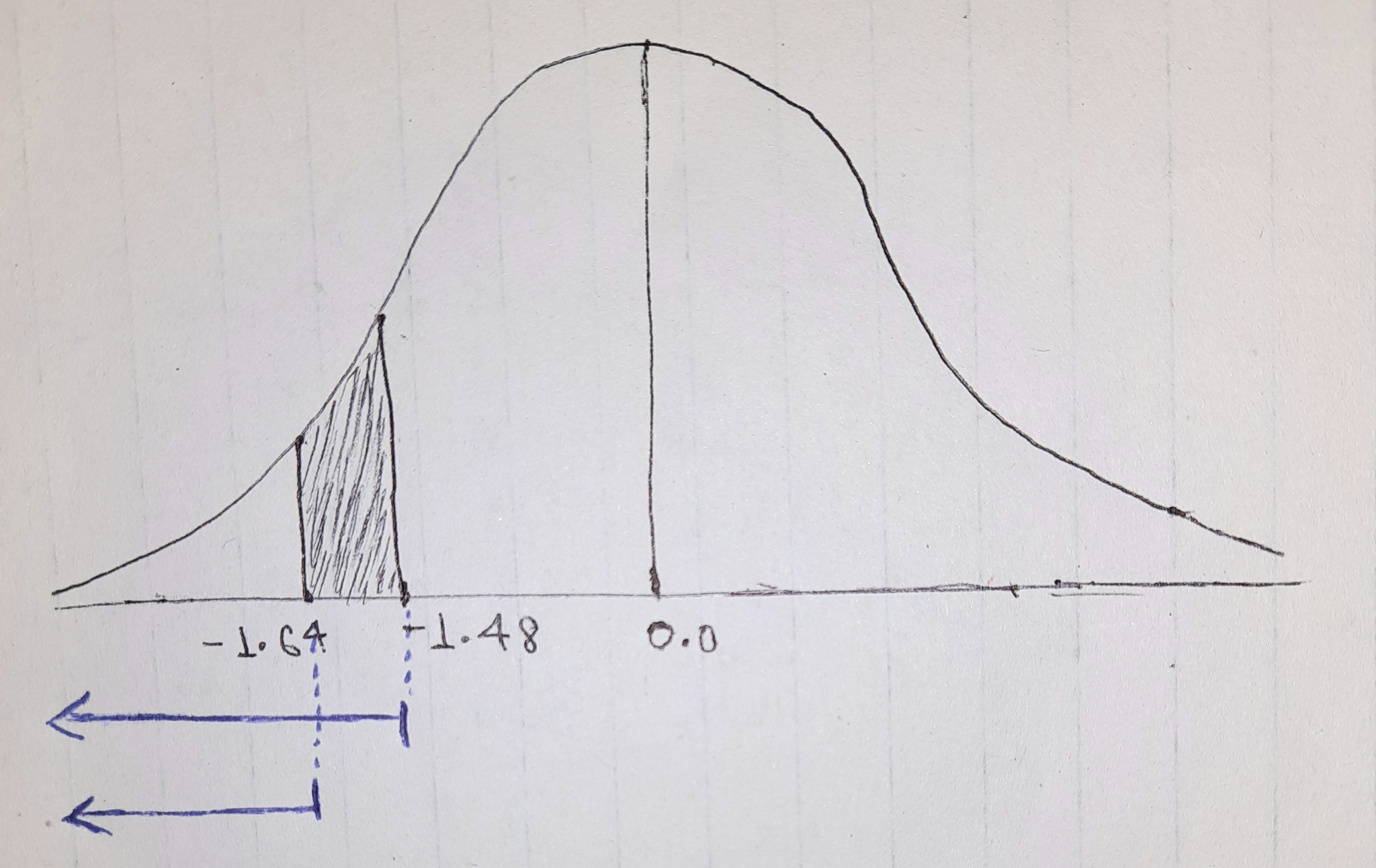So, from the standard normal table, we get: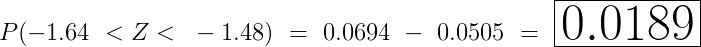NOTE: Remember we have to use the standard normal table in which all Z entries are negative.

==============================================================

METHOD 2:

Now, we will use the standard normal table which all Z positive entries.

So,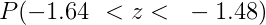This can be written as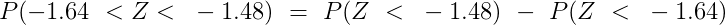Now,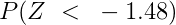can be found using a formula that: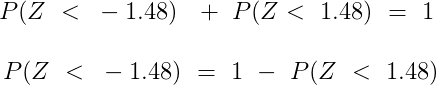(Total area under the curve is equal to 1)

So, from standard normal table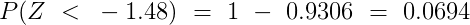Similarly,

we can find: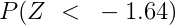So, we get: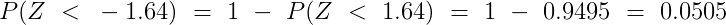Thus, we get: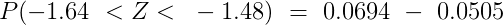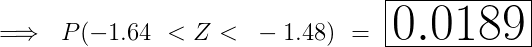==============================================================================

You can use any method which you feel more comfortable.

## Related Solutions

##### Let z be a random variable with a standard normal distribution. Find “a” such that P(|Z|...
Let z be a random variable with a standard normal distribution. Find “a” such that P(|Z| <A)= 0.95 This is what I have: P(-A<Z<A) = 0.95 -A = -1.96 How do I use the symmetric property of normal distribution to make A = 1.96? My answer at the moment is P(|z|< (-1.96) = 0.95
##### Let z be a random variable with a standard normal distribution. Find P(0 ≤ z ≤...
Let z be a random variable with a standard normal distribution. Find P(0 ≤ z ≤ 0.46), and shade the corresponding area under the standard normal curve. (Use 4 decimal places.)
##### For a standard normal distribution, find 1. P(z > c)=0.3796 Find c. 2. P(z < c)=0.0257...
For a standard normal distribution, find 1. P(z > c)=0.3796 Find c. 2. P(z < c)=0.0257 Find c. 3. P(-2.68< z > -0.38) 4. P(z > -1.55) 5. P(z < -0.32)
##### For a standard normal distribution, find: P(z < c) = 0.0414 Find c rounded to two...
For a standard normal distribution, find: P(z < c) = 0.0414 Find c rounded to two decimal places.
##### For a standard normal distribution, find: P(z < -0.64) Express the probability as a decimal rounded...
For a standard normal distribution, find: P(z < -0.64) Express the probability as a decimal rounded to 4 decimal places.
##### Find the following probabilities for the standard normal random variable z z : a) P(−2.07≤z≤1.93)= P...
Find the following probabilities for the standard normal random variable z z : a) P(−2.07≤z≤1.93)= P ( − 2.07 ≤ z ≤ 1.93 ) = (b) P(−0.46≤z≤1.73)= P ( − 0.46 ≤ z ≤ 1.73 ) = (c) P(z≤1.44)= P ( z ≤ 1.44 ) = (d) P(z>−1.57)= P ( z > − 1.57 ) =
##### A) If Z is standard normal, then P(Z < 0.5) is
A) If Z is standard normal, then P(Z < 0.5) is
##### Find the area under the standard normal distribution curve: a) Between z = 0 and z...
Find the area under the standard normal distribution curve: a) Between z = 0 and z = 1.95 b) To the right of z = 1.99 c) To the left of z = -2.09 How would I do this?
##### Find the area under the standard normal distribution curve: a) Between z = 0 and z...
Find the area under the standard normal distribution curve: a) Between z = 0 and z = 1.95 b) To the right of z = 1.99 c) To the left of z = -2.09 How would I do this?
##### QUESTION 5 The variable Z has a standard normal distribution. The probability P(- 0.5 < Z...
QUESTION 5 The variable Z has a standard normal distribution. The probability P(- 0.5 < Z < 1.0) is: a. 0.5328 b. 0.3085 c. 0.8413 d. 0.5794 QUESTION 6 If a random variable X is normally distributed with a mean of 30 and a standard deviation of 10, then P(X=20) = a. 0.4772 b. -0.4772 c. -2.00 d. 0.00 QUESTION 7 If P( -z < Z < +z) = 0.8812, then the z-score is: a. 1.56 b. 1.89 c. 0.80...